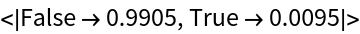Function Repository Resource:

ContainsAllMultiplicities

Similar to ContainsAll but taking the multiplicities into consideration

Contributed by: Sander Huisman
 ResourceFunction["ContainsAllMultiplicities"][e1,e2] yields True if e1 contains all of the elements of e2 taking into account multiplicities. ResourceFunction["ContainsAllMultiplicities"][e2] is an operator form that yields True when the object to which it is applied contains all of the elements of e2.

Examples

Basic Examples

Check if the elements of the second list are all contained in the first list:

 In:=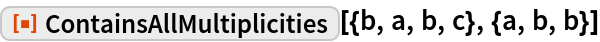Out=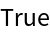Check if the elements of the second list are all contained in the first list:

 In:=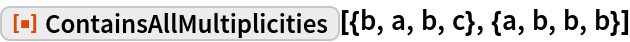Out=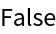Scope

Make a function that checks if a list has one 1, two 2s and one 3:

 In:=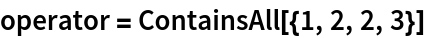Out=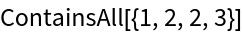Apply to a bunch of lists:

 In:=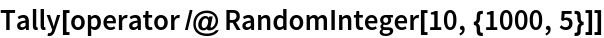Out=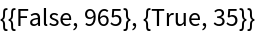Properties and Relations

Elements of e1 and e2 can be lists themselves:

 In:=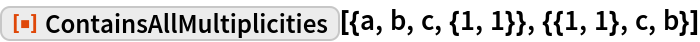Out=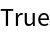There are not enough c’s in the first list:

 In:=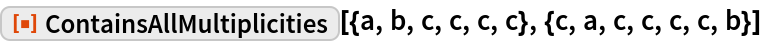Out=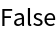Check the same but without considering the multiplicities:

 In:=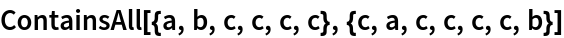Out=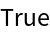If e1 is empty and e2 is non-empty, the answer is always False:

 In:=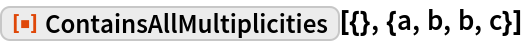Out=If e2 is empty, the answer is always True:

 In:=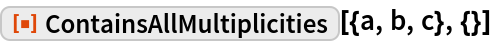Out=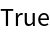If e1 and e2 are empty, the answer is True:

 In:=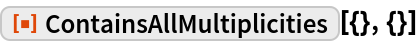Out=Neat Examples

What are the chances that two randomly generated lists of length 10 overlap exactly:

 In:=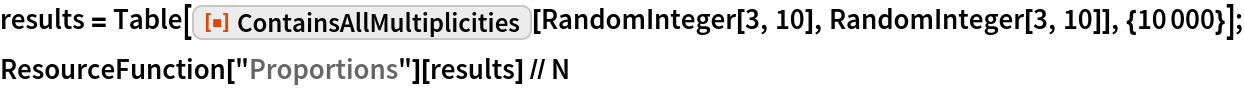Out=